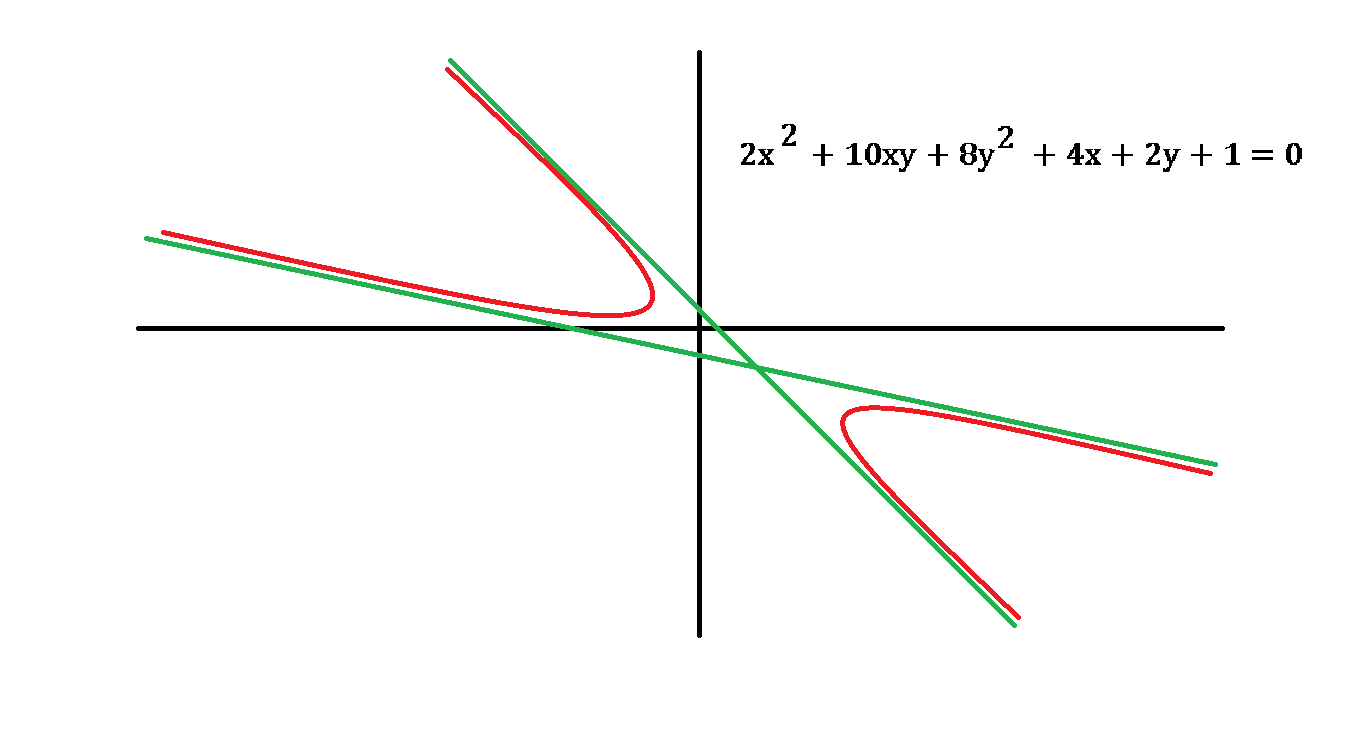# Bizarre Hyperbola

Calculus Level 4The hyperbola above has equation $2x^2 + 10xy + 8y^2 + 4x + 2y + 1 = 0$.

Let $m_{1}$ and $m_{2}$ be the slopes of the asymptotes (shown in green) such that $|m_{1}| > |m_{2}|$, and let $m_{3}$ be the slope of the line that passes through both points, where the tangents have infinite slopes.

Find the value of $\dfrac{1}{|m_{1}| - |m_{2}| - |m_{3}|}$.

×